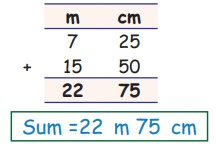Home | | Maths 5th Std | Addition of length

## Chapter: 5th Maths : Term 1 Unit 4 : Measurements

5th Maths : Term 1 Unit 4 : Measurements : Addition of Length

Example

1. Find the sum of the following.

(i) 7 m 25 cm + 15 m 50 cmSum = 22 m 75 cm

Step 1: Start from centimeter

25 cm + 50 cm = 75 cm

7 m + 15 m = 25 m

(ii) 5 km 700 m + 12 km 450mSum = 18 km 150 m

700 + 450 = 1150

Step 2: Convert meter into kilometer

1150 ÷ 1000 = 1 km 150 m

Step 3: Add 1 km to the km column

1 km + 5 km + 12 km = 18 km

1+5+12=18

Example

2. The length of three ropes are 1 m 20 cm, 2 m 15 cm and 1 m 25 cm. What is the total length of three ropes?

Solution:Total length of the ropes = 4 m 60 cm

Tags : Measurements | Term 1 Chapter 4 | 5th Maths , 5th Maths : Term 1 Unit 4 : Measurements
Study Material, Lecturing Notes, Assignment, Reference, Wiki description explanation, brief detail
5th Maths : Term 1 Unit 4 : Measurements : Addition of length | Measurements | Term 1 Chapter 4 | 5th Maths